# NCERT Solutions For Class 10 Maths Chapter 6 Exercise 6.4

## Chapter 6 Ex.6.4 Question 1

Let $$\Delta ABC \sim \Delta DEF$$ and their areas be, respectively, $${64\,\rm{c}}{{\rm{m}}^{\rm{2}}}$$ and $${121\,\rm{c}}{{\rm{m}}^{\rm{2}}}$$. If $$E F=15.4\, \rm{cm}$$ find $$BC.$$

Diagram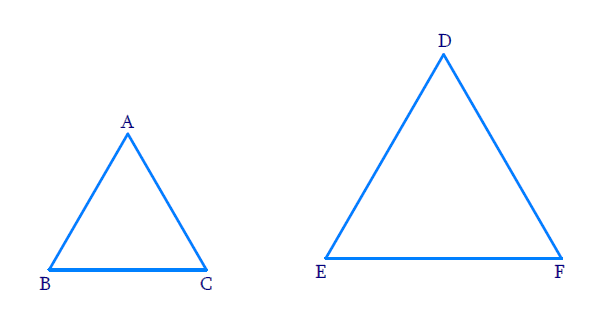### Solution

Reasoning:

As we know that the ratio of the areas of two similar triangles is equal to the square of the ratio of their corresponding sides.

Steps:

$$\Delta ABC \sim \Delta DEF$$

\begin{aligned} \frac{\text { Area of } \Delta A B C}{\text { Area of } \Delta D E F} &=\frac{(B C)^{2}}{(E F)^{2}} \\ \\ \frac{64\, \rm{c m^{2}}}{121\, \rm{c m^{2}}} &=\frac{(B C)^{2}}{(15.4)^{2}} \B C)^{2} &=\frac{(15.4)^{2} \times 64}{121} \\ B C &=\frac{15.4 \times 8}{11} \\ B C &=11.2\, \mathrm{cm} \end{aligned} ## Chapter 6 Ex.6.4 Question 2 Diagonals of a trapezium \(ABCD with $$AB || DC$$ intersect each other at the point $$O.$$ If $$AB = 2 \,CD,$$ find the ratio of the areas of triangles $$AOB$$ and $$COD.$$

Diagram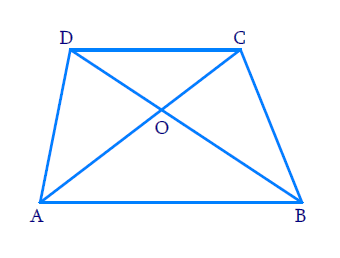### Solution

Reasoning:

The ratio of the areas of two similar triangles is equal to the square of the ratio of their corresponding sides.

$$AA$$ criterion.

Steps:

In trapezium $$ABCD,$$  $$AB\parallel CD\,$$ and $$AB = 2\,CD$$

Diagonals $$AC, BD$$ intersect at ‘$$O$$

In $$\Delta AOB\,\text{and}\,\Delta COD$$

$$\angle AOB = \angle COD$$

(vertically opposite angles)
$$\angle ABO = \angle CDO$$

(alternate interior angles)
$$\Rightarrow \; \Delta AOB \sim \Delta COD$$

(AA criterion)
\begin{align}\Rightarrow \frac{{{\rm{Area \,of\,}}{{\Delta AOB}}}}{ \text{Area} \,of \Delta COD} & = \frac{{{{(AB)}^2}}}{{{{(CD)}^2}}} \\ & (\text{Theorem} \, 6.6) \end{align}

\begin{align} \qquad =\frac{{{{(2CD)}^2}}}{{{{(CD)}^2}}}&=\frac{{4C{D^2}}}{{C{D^2}}} = \frac{{4}}{1}\end{align}

\begin{align} \Rightarrow \; &\text{Area of } \Delta AOB : \text{Area of } \Delta COD \\=\;& 4:1 \end{align}

## Chapter 6 Ex.6.4 Question 3

In Fig. below, $$ABC$$ and $$DBC$$ are two triangles on the same base $$BC.$$ If $$AD$$ intersects $$BC$$ at $$O,$$ show that \begin{align}\frac{{ar\Delta ABC}}{{ar\Delta DBC}} = \frac{{AO}}{{DO}}\end{align}

Diagram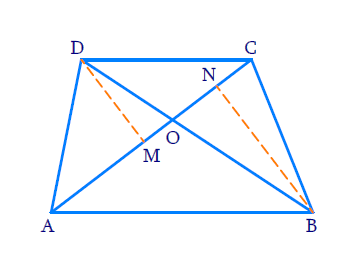### Solution

Reasoning:

AA criterion

Steps:

In $$\,\Delta ABC$$

Draw $$\,AM \bot BC$$

In $$\,\,\Delta DBC$$

Draw $$\,DN \bot BC$$

Now in $$\,\,\Delta AOM,\,\,\Delta DON$$

\begin{align} &\angle AMO=\angle D N O=90^{\circ} \\ &\angle A O M= \angle DON \\ &\text{(Vertically opposite angles) } \end{align}

\begin{align}\Rightarrow \quad &\Delta AOM \sim \Delta DON \\ &\text{(AA Criterion)}\end{align}

\begin{align} {\Rightarrow \quad\frac{A M}{D N}}={\frac{O M}{O N}=\frac{A O}{D O} \ldots (1)}\end{align}

Now,

\begin{align} ar\Delta ABC& \! = \! \frac{1}{2} \! \times \! \text{Base} \! \times \! \text{Height}\\& \! = \! \frac{1}{2} \! \times \! BC \! \times \! AM \\ ar\Delta DBC& \! = \! \frac{1}{2} \! \times \! BC \! \times \! DN \\\frac{ar \Delta ABC}{ ar\Delta DBC}& \! = \! \frac{\frac{1}{2} \! \times \! BC \! \times \! AM}{\frac{1}{2} \! \times \! BC \! \times \! DN}\\\frac{ ar\Delta ABC}{ ar \Delta DBC}& \! = \! \frac{AM}{DN}\\\frac{ ar\Delta ABC}{ ar\Delta DBC}& \! = \! \frac{AO}{DO} \qquad \dots\text{from} (1) \end{align}

## Chapter 6 Ex.6.4 Question 4

If the areas of two similar triangles are equal, prove that they are congruent.

Diagram### Solution

Reasoning:

As we know that two triangles are similar if their corresponding angles are equal and their corresponding sides are in the same ratio.

The ratio of the areas of two similar trianglesis equal to the square of the ratio of their corresponding sides.

As we know if three sides oh one triangle are equal to the three sides of another triangle,then the two triangles are congruent.

Steps:

$$\Delta ABC \sim \Delta DEF$$

\begin{align}\Rightarrow \quad&\frac{{AB}}{{DE}} = \frac{{BC}}{{EF}} = \frac{{CA}}{{FD}}\\ & \text{(SSS Criterion)} \end{align}

\begin{align}&\;ar\Delta ABC=\Delta DEF\\\Rightarrow\quad&\frac{{ar\Delta ABC}}{{ ar\Delta DEF}} = 1\;\;\dots(1)\end{align}

But,

\begin{align}\frac{{ar\Delta ABC}}{{ar\Delta DEF}} &= \frac{{{{(AB)}^2}}}{{{{(DE)}^2}}} \\= \frac{{{{(BC)}^2}}}{{{{(EF)}^2}}} &= \frac{{{{(CA)}^2}}}{{{{(FD)}^2}}}\end{align}

From $$(1)$$

\begin{align}{\frac{(A B)^{2}}{(D E)^{2}}}&=\frac{(B C)^2}{(E F)^2} \\ & =\frac{(C A)^2}{(F D)^2} \\ & =1 \\ {\Rightarrow \; \frac{(A B)^{2}}{(D E)^{2}}}&={1} \\ {\Rightarrow \;\; (AB)^{2}}&={(D E)^{2} }\\ \Rightarrow \;\;\quad A B &=DE \;\;\ldots (2)\end{align}

Similarly,

$$\Rightarrow$$   $$BC = EF .... (3)$$

$$\Rightarrow$$   $$CA =FD …. (4)$$

Now, in $$\Delta ABC,\,\Delta DEF$$

$$\Rightarrow$$$$AB = DE$$    $$\text{(from 2)}$$

$$\Rightarrow$$$$BC = EF$$    $$\text{(from 3)}$$

$$\Rightarrow$$$$CA = FD$$    $$\text{(from 4)}$$

\begin{align} \Rightarrow\quad &\Delta ABC \cong \Delta DEF\\& \text{(SSS congruency)}\end{align}

## Chapter 6 Ex.6.4 Question 5

$$D, E$$ and $$F$$ are respectively the mid-points of sides $$AB, BC$$ and $$CA$$ of $$\Delta \,ABC.$$ Find the ratio of the areas of $$\Delta \,DEF$$ and $$\Delta \,ABC.$$

Diagram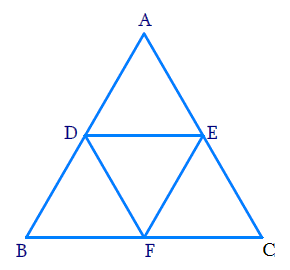### Solution

Reasoning:

As we know that the line segment joining the midpoints of two sides of a triangle is parallel to the third side and half of it – (mid-point theorem).

Steps:

In $$\Delta ABC$$, $$D\, {\rm{}and}\, F$$ are the midpoints of $$AB, AC$$ respectively.

\begin{align} \Rightarrow \;\;&DE\parallel BC \;\text{ and }\; DE = \frac{1}{2}BC \\ &\text{(by mid-point theorem)} \end{align}

Again, $$E$$ is mid-point of $$BC$$

\begin{align} \Rightarrow \;\;DF \parallel BE \;\text{ and }\; DF = BE\end{align}

In quadrilateral $$DFEB,$$

\begin{align} DF \parallel BE \;\text{ and }\; DF = BE\end{align}

$$\therefore$$ $$DFEB$$ is a parallelogram

\begin{align}\Rightarrow \quad &\angle B = \angle F\;\;\dots(1) \\ &\begin{bmatrix} \text{Opposite angles of a } \\ \text{parallelogram are equal}\end{bmatrix} \end{align}

Similarly, we can prove that

$$DFCE$$ is a parallelogram

\begin{align}\Rightarrow \quad& \angle C=\angle D\;\;\dots(2)\\ &\begin{bmatrix} \text{Opposite angles of a } \\ \text{parallelogram are equal}\end{bmatrix} \end{align}

Now,

In $$\Delta DEF$$ and $$\Delta ABC$$

$\angle DEF = \angle ABC \;\;\dots \text{from (1)}$

$\angle EDF = \angle ACB \;\;\dots \text{from (2)}$

\begin{align}\Rightarrow \quad & \Delta DEF \sim \Delta CAB \\ & \text{ [AA Criterion]} \end{align}

The ratio of the areas of two similar triangles is equal to the square of corresponding sides.

\begin{align}\frac{{ \text{ar}\,\Delta DEF}}{{\text{ar}\,\Delta ABC}} & = \frac{{{{(DE)}^2}}}{{{{(AC)}^2}}} \\ & = \frac{{{{(EF)}^2}}}{{{{(AB)}^2}}} \\ & = \frac{{{{(DF)}^2}}}{{{{(BC)}^2}}}\end{align}

\begin{align} \frac{\text {ar} \Delta D E F}{\text {ar} \Delta A B C} &=\frac{(D F)^{2}}{(BC)^{2}} \\ &=\frac{(\frac12 BC)^{2}}{(BC)^{2}} \quad\\ &=\frac{B C^{2}}{4 B C^{2}}\\ &=\frac{{1}}{4} \end{align}

The ratio of the areas of $$\Delta DEF$$ and $$\Delta ABC$$ is $$1:4$$

Alternate  method:

Reasoning:

Mid-Point Theorem : The line segment joining the mid-points of two sides of a triangle is parallel to the third side.

Steps:

In $$\Delta ABC$$ ,$$D$$ and $$E$$ are midpoints of sides $$AB$$ and $$AC$$

$\Rightarrow DE\parallel BC$

and

\begin{align} DE \!=\! \frac{1}{2}BC \end{align} \dots (1)

Now in quadrilateral $$DBFE$$

$\Rightarrow DE\parallel BC \text{ and }DE \!=\! BF \dots\text{from (1)}$

$$\Rightarrow$$ $$DBFE$$ is a parallelogram

\begin{align} &\Rightarrow \quad ar\Delta DBF \!=\! ar \Delta DEF \;\;\dots(2) \\ &\begin{bmatrix}\because \text{Diagonal DF divides the parallelogram } \\ \text{into two triangles of equal area} \end{bmatrix}\end{align}

Similarly, we can prove

$ar\Delta DBF \! = \! ar\Delta EFC\;\; \dots (3)$

$ar\Delta DEF \! = \! ar\Delta ADE\;\; \dots (4)$

From $$(2 )$$ $$( 3)$$ and $$(4)$$

$\begin{bmatrix}ar\Delta DBF \! = \! ar\Delta DEF \! =\\ \! ar\Delta EFC \! = \! ar\Delta ADE\end{bmatrix}\;\;\dots(5)$

Things which are equal to the same thing are equal to one another $$–$$ Euclid’s $$1$$st axiom.

\begin{align} \begin{bmatrix} ar\Delta ABC = ar\Delta ADE = ar\Delta DBF = \\ar\Delta EFD = ar\Delta DEF \end{bmatrix} \end{align}

From $$(5)$$

\begin{align} ar\Delta ABC & \! = \! 4 \! \times \! ar\Delta DEF \\\frac{ar\Delta DEF}{ar\Delta ABC} & \! = \! \frac{1}{4}\\ar\Delta DEF : ar\Delta ABC & \! = \! 1 \! : \! 4 \end{align}

## Chapter 6 Ex.6.4 Question 6

Prove that the ratio of the areas of two similar triangles is equal to the square of the ratio of their corresponding medians.

Diagram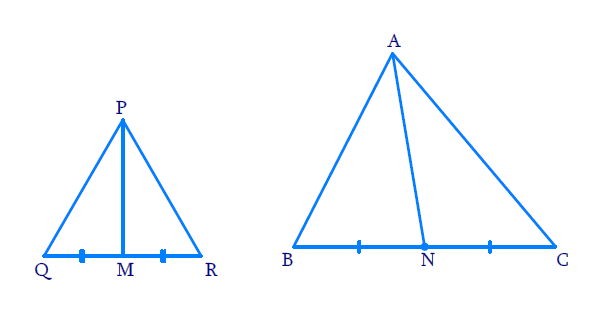### Solution

Reasoning:

As we know,if one angle of a triangle is equal to one angle of the other triangle and the sides including these angles are proportional, then the two triangles are similar.And we know that the ratio of the areas of two similar triangles is equal to the square of the ratio of their corresponding sides.

Steps :

In $$\Delta PQR$$, $$PM$$ is the median and, in $$\Delta ABC$$ $$AN$$ is the median

$\Delta P Q R \sim \Delta ABC\;\;\dots(\text {Given})$

\begin{align} &\angle PQR \! = \! \angle ABC \;\; \dots\left( 1 \right) \\ & \angle QPR \! = \! \angle BAC \;\; \dots\left( 2 \right) \\ & \angle QRP \! = \! \angle BCA \;\; \dots\left( 3 \right)\\\\ & \qquad\qquad \text{and} \\\\ &\frac{PQ}{AB} \! = \! \frac{QR}{BC} \! = \! \frac{RP}{CA} \;\; \dots(4)\end{align}

($$\because$$ If two triangles are similar, then their corresponding angles are equal andcorresponding sides are in the same ratio)

\begin{align}\frac{ar\Delta PQR}{ar\Delta ABC} & \! = \! \frac{{{{(PQ)}^2}}}{{{{(AB)}^2}}} \\ & \! = \! \frac{{{{(QR)}^2}}}{{{{(BC)}^2}}} \\ & \! = \! \frac{{{{(RP)}^2}}}{{{{(CA)}^2}}}\\ &[\text{from Theorem 6.6}] \end{align} \\ \dots(5)

Now, In $$\Delta PQM$$ and $$\Delta ABN$$

$\angle PQM \! = \! \angle ABN \;\;\dots \text{from (1)}$

And \begin{align}\frac{{PQ}}{{AB}} = \frac{{QM}}{{BN}}\end{align}

\begin{align} \frac{PQ}{AB} = \frac{QR}{BC} = \frac{2QM}{2BN} \end{align}

$$M,N$$ are mid points of $$QR$$ and $$BC$$

$\Rightarrow \Delta PQM \sim \Delta ABN$

[$$\rm SAS$$ similarity]

\begin{align} \Rightarrow \,\,\frac{ar\Delta PQM}{ar\Delta ABN} & = \frac{(PQ)^2}{(AB)^2} \\ & = \frac{(QM)^2}{(BN)^2} \\ & =\frac{(PM)^2}{(AN)^2} \\ &\dots[\text{Theorem 6.6}] \end{align}

from $$(5)$$ and $$(6)$$

\begin{align}\frac{ar\Delta PQR}{ar\Delta ABC}=\frac{{{(PM)}^{2}}}{{{(AN)}^{2}}}\end{align}

## Chapter 6 Ex.6.4 Question 7

Prove that the area of an equilateral triangle described on one side of a square is Equal to half the area of the equilateral triangle described on one of its diagonals.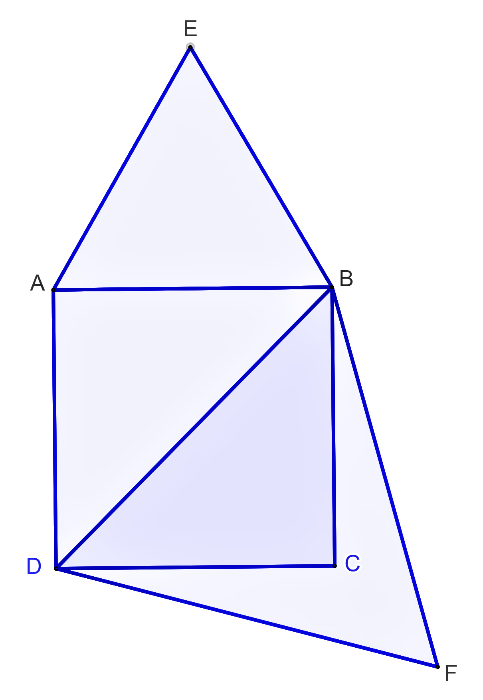### Solution

Reasoning:

As we know that the ratio of the areas of two similar triangles is equal to the square of the ratio of their corresponding sides.

Steps:

$$\Delta ABE\,\,$$ is described on the side $$AB$$ of the square $$ABCD$$

$$\Delta DBF$$ is described on the diagonal $$BD$$ of the square $$ABCD$$

Since $$\Delta ABE\,$$ and $$\,\Delta DBF$$ are equilateral triangles

$$\Delta ABE \sim \Delta DBF$$ [each angle in an equilateral triangle measures $${{60}^ \circ}$$ ]

The ratio of the areas of two similar triangles is equal to the square of the ratio of the corresponding sides.

\begin{align}&\frac{{ar\Delta ABE}}{{ar\Delta DBF}}= \frac{{{{(AB)}^2}}}{{{{(DB)}^2}}} \\&\frac{{ar\Delta ABE}}{{ar\Delta DBF}}= \frac{{{{\left( {AB} \right)}^2}}}{{{{\left( {\sqrt 2 AB} \right)}^2}}}\\& [\therefore \; \text{Diagonal of a square is } \sqrt 2\times\text{side} ] \end{align}

\begin{align}&\frac{{ar\Delta ABE}}{{ar\Delta DBF}}= \frac{{A{B^2}}}{{2A{B^2}}}\\&\frac{{ar\Delta ABE}}{{ar\Delta DBF}} = \frac{1}{2}\\\Rightarrow \quad &\;\;ar\Delta ABE = \frac{1}{2} ar\Delta DBF\end{align}

Tick the correct answer and justify.

## Chapter 6 Ex.6.4 Question 8

$$ABC$$ and $$BDE$$ are two equilateral triangles such that $$D$$ is the mid-point of $$BC.$$ Ratio of the areas of triangles $$ABC$$ and $$BDE$$ is

(a) $$2 : 1$$

(b) $$1 : 2$$

(c) $$4 : 1$$

(d) $$1 : 4$$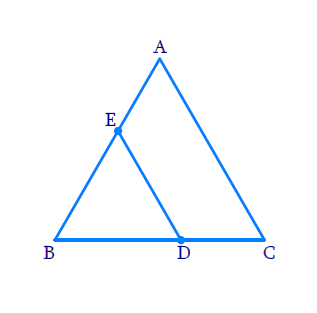### Solution

Reasoning:

$$AAA$$ criterion.

Steps:

$$\Delta A B C \sim \Delta B D E$$ $$(\because \text{equilateral triangles)}$$

The ratio of the areas of two similar triangles is equal to the square of the ratio of their corresponding sides.

\begin{aligned} \frac{ar\Delta A B C}{ar \Delta B D E} &=\frac{(B C)^{2}}{(B D)^{2}} \\ &=\frac{(B C)^{2}}{\left(\frac{B C}{2}\right)^{2}} \\ [ D \text { is the} & \text{ mid point} \text{ of } BC] \\ &=\frac{(B C)^{2} \times 4}{(B C)^{2}}\\&=4 \end{aligned}

\begin{aligned} ar\Delta ABE : ar\Delta BDE =4:1\end{aligned}

The answer is (c) $$4:1$$

## Chapter 6 Ex.6.4 Question 9

Sides of two similar triangles are in the ratio $$4 : 9.$$ then the areas of these triangles are in the ratio

(a) $$2: 3$$

(b) $$4 : 9$$

(c) $$81 : 16$$

(d) $$16 : 81$$

### Solution

Reasoning:

The ratio of the areas of two similar triangles is equal to the square of the ratio of their corresponding sides.

Steps:

We know that,

The ratio of the areas of two similar triangles is equal to the square of the ratio of their corresponding sides.

$= {(4\;:\;9)^2}\\= 16 : 81$

The answer is (d) $$16 : 81$$

Download SOLVED Practice Questions of NCERT Solutions For Class 10 Maths Chapter 6 Exercise 6.4 for FREE
Ncert Class 10 Exercise 6.4
Ncert Solutions For Class 10 Maths Chapter 6 Exercise 6.4
Instant doubt clearing with Cuemath Advanced Math Program